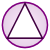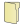Games
Problems
Go Pro!

# Analytic Geometry

Pro Problems > Math > Geometry > Analytic Geometry

# Featured Pro ProblemsFind the equation of a line that bisects the angle between two other linesFind the equations of the circle and the parabola based on the information givenFind the perimeter of a triangle, given the equations of the lines that form its sidesA circle is sandwiched between two lines. Find all possible equationsFind the equation of the parabola, based on the coordinates of three points which lie on the parabolaFind the radius of a second circle, given information about how it intersects with the first circleMake a triangle right, by correctly placing a vertex on the Cartesian PlaneFind the radii of two circles which have their centers on the latus rectum of a parabola# Full Directory Listing

Ellipse and HyperbolaLike us on Facebook to get updates about new resources